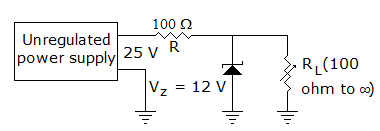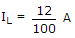# Electronics and Communication Engineering - Analog Electronics - Discussion

11.

In figure The minimum and maximum load currents are[A]. 0 and 60 mA [B]. 0 and 120 mA [C]. 10 mA and 60 mA [D]. 10 mA and 120 mA

Explanation:

When RL = ∞, IL = 0,

When RL = 100 Ω,or 120 mA.

 Sandeep said: (Jan 26, 2015) What is 25v?

 Paul said: (Mar 31, 2015) Why doesn't the first 100 ohm resistor add to the series resistance?

 Babroo Chavan said: (Apr 14, 2017) Why only 12 volt used here. Then What is 25v?

 Reshma said: (Apr 19, 2017) Here zener diode is coming parallel to load.

 Maharaja said: (May 7, 2017) Why particularly use 12v in load current?

 Anandu said: (Jul 5, 2017) I think since it's an unregulated power supply, 25 V tend to fluctuate. A Zener diode in reverse config offers no impedance above its breakdown voltage, in this case, 12V. So a maximum of 12V can be found across that Zener diode which is parallel to RL(thus the same voltage across RL also).

 Waseem Haider said: (Sep 10, 2017) 25v is supply voltges. Zener regulates these volts. 12v zener means it regulates 12volts. So load volt is 12v. So we use ohms law formula I=V/R.

 Wasif said: (Jan 6, 2019) What if, the unregulated power supply will be replaced with the regulated power supply?

 Sonu Kumar Sahani said: (Jan 17, 2020) In ON state, the voltage across load is equal to Zener voltage.